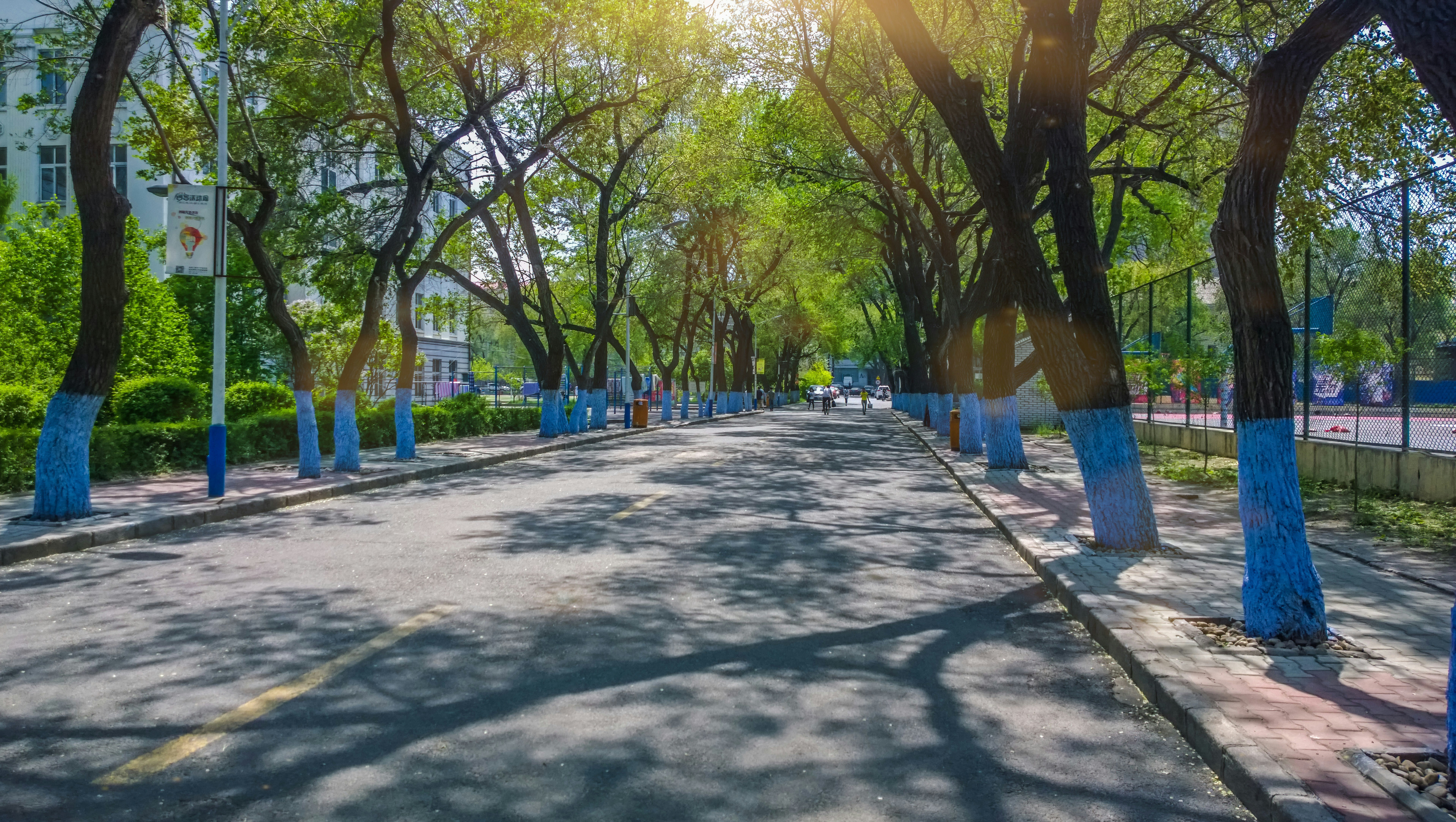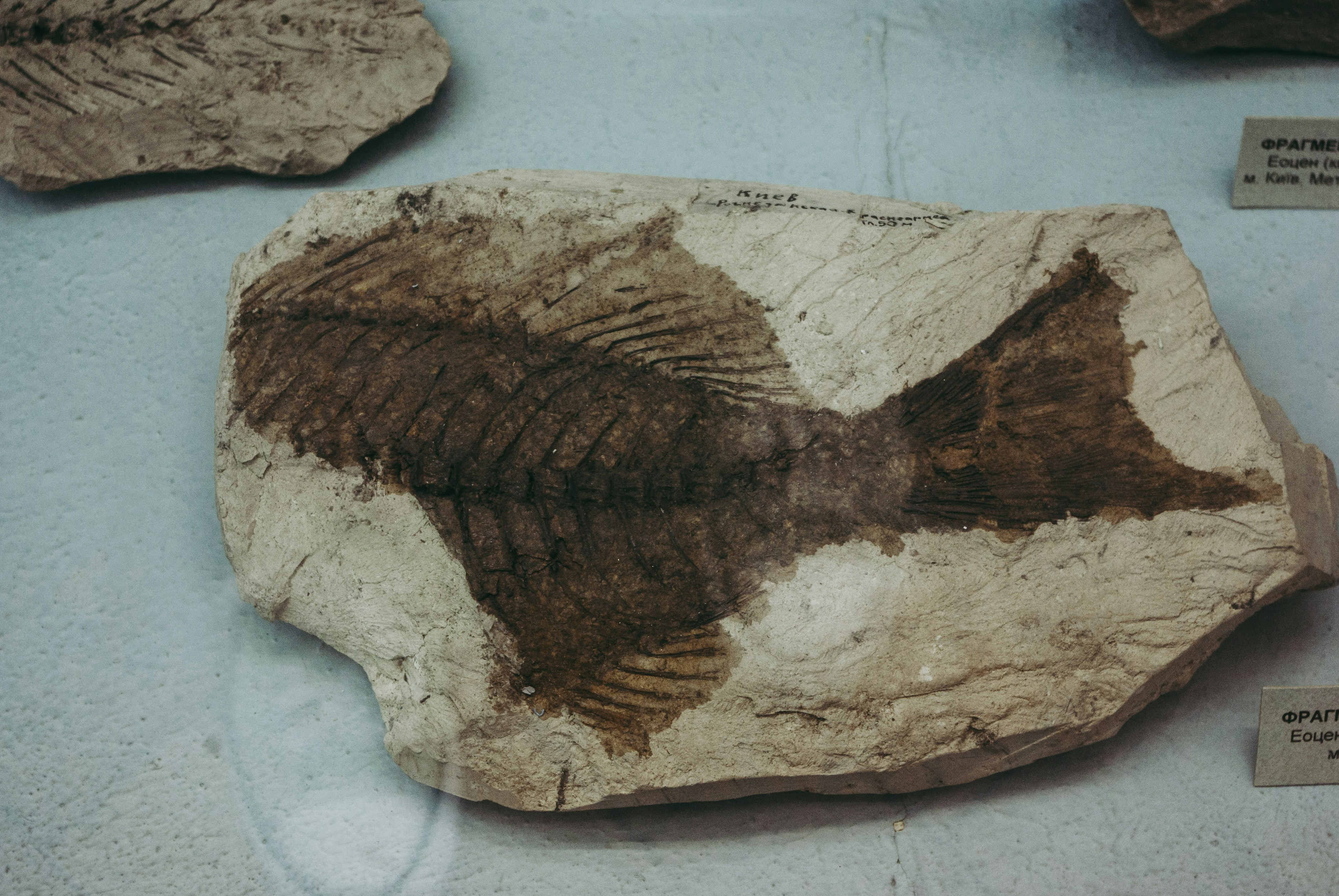Recently PublishedJournal article

## On Classes of Neighborhood Resolving Sets of a GraphJournal article

## On Topological Integer Additive Set-labeling of Star GraphsJournal article

## On Maximum Signless Laplacian Estrada Index of Graphs with Given Parameters II

Most ViewedJournal article

## The Eccentric-distance Sum of Some Graphs

Let $G = (V,E)$ be a simple connected graph. Theeccentric-distance sum of $G$ is defined as$\xi^{ds}(G) =\ds\sum_{\{u,v\}\subseteq V(G)} [e(u)+e(v)] d(u,v)$, where $e(u)$ %\dsis the eccentricity of the vertex $u$ in $G$ and $d(u,v)$ is thedistance between $u$ and $v$. In this paper, we establish formulaeto calculate the eccentric-distance sum for some graphs, namelywheel, star, broom, lollipop, double star, friendship, multi-stargraph and the join of $P_{n-2}$ and $P_2$.Journal article

## Inverse Graphs Associated with Finite Groups

Let $(\Gamma,*)$ be a finite group and $S$ a possibly empty subset of $\Gamma$ containing its non-self-invertible elements. In this paper, we introduce the inverse graph associated with $\Gamma$ whose set of vertices coincides with $\Gamma$ such that two distinct vertices $u$ and $v$ are adjacent if and only if either $u * v\in S$ or $v * u\in S$. We then investigate its algebraic and combinatorial structures.
Suggested For YouJournal article

## Routed Planar Networks

Modeling a road network as a planar graph seems very natural. However, in studying continuum limits of such networks it is useful to take {\em routes} rather than {\em edges} as primitives. This article is intended to introduce the relevant (discrete setting) notion of {\em routed network} to graph theorists. We give a naive classification of all 71 topologically different such networks on 4 leaves, and pose a variety of challenging research questions.